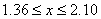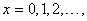Types of variables

Definition: A variable is a characteristic that changes or varies over time and/or for different individuals or objects under consideration.

Variables can be classified into one of two categories: qualitative or quantitative.

Definition: A qualitative variable measures a quality or characteristic of an object

A quantitative variable measures a numerical quantity of an object

Qualitative variables produce data that can be categorized according to similarities or differences in quality. They are usually called categorical data. Variables such as type of car or species of animal are qualitative.

Quantitative variables produce numerical data. The numerical value assigned to a quantitative variable is usually denoted by x,

e.g. x = height of student or x = number of people in a theatre audience.

It will be noted that there is a difference in the types of numerical values that quantitative variables can take. The height of a student can take any value between the smallest height and the largest height of any student measured. If the smallest height is 1.36m and the largest 2.10m, the value for the height can take on any value between 1.36m and 2.10m, which is written as. The height is said to be a continuous variable. However, the number of people in a theatre audience can only be positive whole numbers (including zero if there is no-one in the audience). This is written asIn this case, x is said to be a discrete variable.

Definition: A continuous variable can assume an infinite number of values within a prescribed interval

A discrete variable can only assume a finite, countable number of values within a prescribed interval